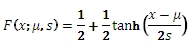# SQL Server LaPlace inverse distribution function

LAPLACEINV

Updated: 31 July 2010

Use LAPLACEINV to calculate the inverse lower cumulative distribution of the Laplace distribution.

The formula for the lower cumulative distribution function is:Syntax
SELECT [wctStatistics].[wct].[LAPLACEINV] (
<@P, float,>
,<@A, float,>
,<@B, float,>)
Arguments
@P
is the value of the lower cumulative distribution function. @P is an expression of type float or of a type that implicitly converts to float
@A
is the location parameter. @A is an expression of type float or of a type that implicitly converts to float
@B
is the scale parameter. @B is an expression of type float or of a type that implicitly converts to float
Return Types
float
Remarks
·         @B must be greater than zero (B > 0).
·         @P must be greater than zero and less than one (0 < @P < 1).
Examples
Calculate the inverse of the lower cumulative distribution function:
SELECT wct.LAPLACEINV(0.696734670143683,0,1)
This produces the following result
----------------------
0.499999999999999

(1 row(s) affected)

### SupportCopyright 2008-2021 Westclintech LLC         Privacy Policy        Terms of Service